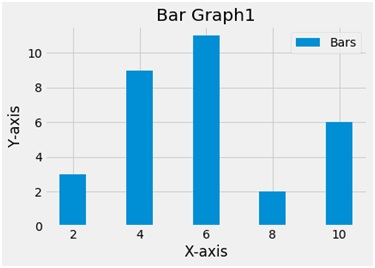# Python | Create a bar chart using matplotlib.pyplot

Here, we are implementing a python program to create a bar char using matplotlib.pyplot.
Submitted by Ayush Sharma, on November 19, 2018

Problem statement: Write a python program using matplotlib.pyplot library to create a bar chart.

A bar chart or bar graph is a chart or graph that presents categorical data with rectangular bars.

Program:

```import matplotlib.pyplot as plt

x = [2,4,6,8,10]
y=[3,9,11,2,6]
plt.bar(x,y,label ='Bars')

plt.xlabel('X-axis')
plt.ylabel('Y-axis')
plt.title('Bar Graph1')
plt.legend()
plt.show()
```

OutputExplanation:

Python library matplotlib.pyplot is used to draw the above chart. Two random variables x and y are taken with random values. The bar function plots a bar plot. The bar function takes 2 arguments i.e. x and y and a label variable gives the label to the plot. To name the axes xlabel and ylabel functions are used and to give the title to the plot the title function is used. To show the legend the legend function is used and finally to show the plot the show function.

Preparation